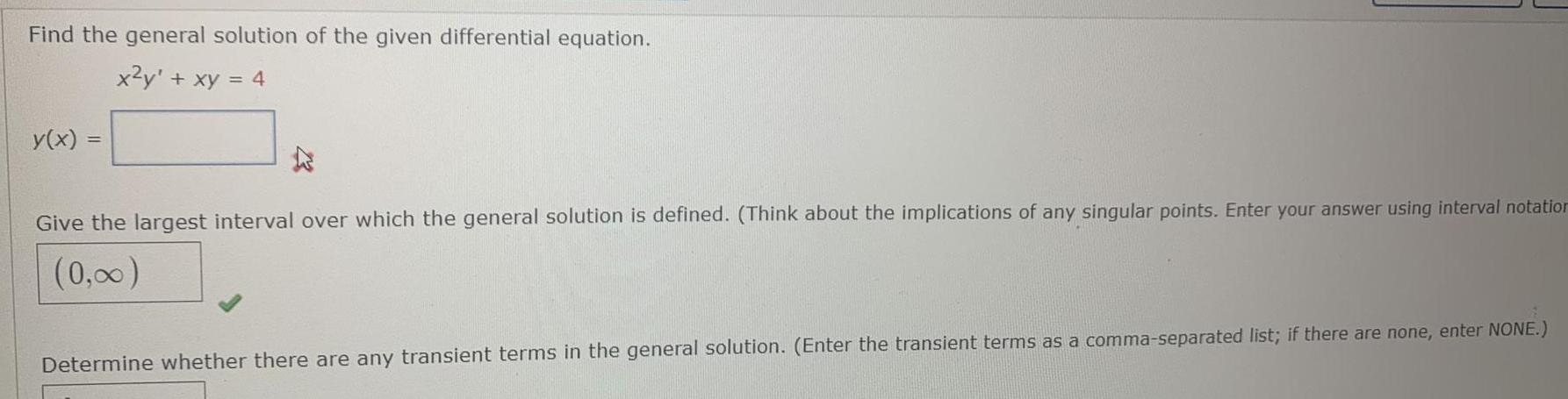Question:

# Find the general solution of the given differential equation. x²y + xy = 4 y(x) = Give the largest interval over which the general solution is defined. (Think about the implications of any singular points. Enter your answer using interval notation

Last updated: 8/15/2022Find the general solution of the given differential equation. x²y + xy = 4 Give the largest interval over which the general solution is defined. (Think about the implications of any singular points. Enter your answer using interval notation) Determine whether there are any transient terms in the general solution. (Enter the transient terms as a comma-separated list; if there are none, enter NONE.)